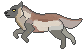(See All?) Announcements
89 Users Online

Vaken vs Sphinx
Print · · Subscribe · 0 Loves ·
@Sphinx versus Vaken. The fight is happening here: https://relic-lore.net/showthread.php?tid=18139&page=2

Vaken's life points = 680 (health = 80%)
stats:
HP- 26
DEF- 13
ATK- 9
DMG- 6
IN- 4

Sphinx life points = 330 (health = 100%)
HP- 29
DEF- 9
ATK- 2
DMG- 6
IN- 2

Vaken will be attacking in the next post. We'd like all the dice rolls once Ashbash agrees! Thank you :)
I give consent!
Code:
`[attacker=“Vaken” HP=“26” DEF=“13” ATK=“9” DMG=“6” IN=“4”][defender=“Sphinx” HP=“29” DEF=“9” ATK=“2” DMG=“6” IN=“2”]`

Roll Results:
“Vaken” rolls a d15 and gets a 13.
“Vaken”'s' ATK: “9”
“9” + 13 = 22

“Sphinx”'s DEF: “9”
22 > “9”

The attack was a HIT!

“Vaken” rolls a d6 and gets a 2.
“Vaken”'s DMG: “6”
“6” + 2 = 8

“Sphinx”'s HP: “29”
“29” - 8 = 21

“Sphinx”'s HP: 21
“Vaken”'s HP: “26”It is Sphinx turn now! Is it possible to get all the rolls at once? Thank you!currently travelling with Circe (she can jump in any of his threads)Code:
`[attacker="Sphinx" HP="21" DEF="9" ATK="2" DMG="6" IN="2"][defender="Vaken" HP="26" DEF="13" ATK="9" DMG="6" IN="4"]`

Roll Results:
Sphinx rolls a d15 and gets a 13.
Sphinx's' ATK: 2
2 + 13 = 15

Vaken's DEF: 13
15 > 13

The attack was a HIT!

Sphinx rolls a d6 and gets a 4.
Sphinx's DMG: 6
6 + 4 = 10

Vaken's HP: 26
26 - 10 = 16

Vaken's HP: 16
Sphinx's HP: 21Code:
`[attacker="Vaken" HP="16" DEF="13" ATK="9" DMG="6" IN="4"][defender="Sphinx" HP="21" DEF="9" ATK="2" DMG="6" IN="2"]`

Roll Results:
Vaken rolls a d15 and gets a 6.
Vaken's' ATK: 9
9 + 6 = 15

Sphinx's DEF: 9
15 > 9

The attack was a HIT!

Vaken rolls a d6 and gets a 5.
Vaken's DMG: 6
6 + 5 = 11

Sphinx's HP: 21
21 - 11 = 10

Sphinx's HP: 10
Vaken's HP: 16Code:
`[attacker="Sphinx" HP="10" DEF="9" ATK="2" DMG="6" IN="2"][defender="Vaken" HP="16" DEF="13" ATK="9" DMG="6" IN="4"]`

Roll Results:
Sphinx rolls a d15 and gets a 10.
Sphinx's' ATK: 2
2 + 10 = 12

Vaken's DEF: 13
12 < 13

The attack was a MISS!

Vaken's HP: 16
Sphinx's HP: 10Code:
`[attacker="Vaken" HP="16" DEF="13" ATK="9" DMG="6" IN="4"][defender="Sphinx" HP="10" DEF="9" ATK="2" DMG="6" IN="2"]`

Roll Results:
Vaken rolls a d15 and gets a 15.
Vaken's' ATK: 9
9 + 15 = 24

Sphinx's DEF: 9
24 > 9

The attack was a CRITICAL HIT!

Vaken rolls a d6 and gets a 2.
Vaken's DMG: 6
6 + 2 = 8

Sphinx's HP: 10
10 - 8 = 2

Sphinx's HP: 2
Vaken's HP: 16At this point I would like permission from @Sphinx to continue because if Vaken rolls a full damage of 6 on his next hit that would put Sphinx at -10 which would make her dead.No need to roll again since we now know Vaken wins so Sphinx would surrender at this point